# How to Use The Excel UNIQUE Function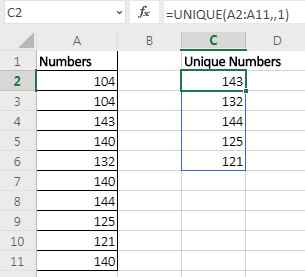Earlier in Excel 2016 and older versions we used complex formulas to extract unique values from a range. In Excel 365 online version, MS provides a new dynamic array function UNIQUE that simply returns the uniques from a given list.

Syntax of UNIQUE Function

=UNIQUE(array,[by_col],[exactly_once])

Array: The array from which you want to extract unique values:

[by_col]: Set it TRUE(1) if the array is horizontal. By default it is FALSE for vertical data.

[exactly_once]: set it TRUE(1) if you want extract values that occur only once in the array. By default it is FALSE(0) to extract all unique values.

Let us have some examples to understand how this UNIQUE function works.
Example of UNIQUE Function
Example 1: Extract unique numbers from the list using UNIQUE function of Excel 365.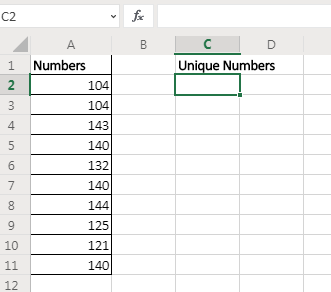Here I have sample data. In range A2:A11 I have some numbers that contain duplicate values. I want to get unique values from this range. I will simply use the UNIQUE function of Excel 365.

=UNIQUE(A2:A11)

This formula simply returns all unique values from the range.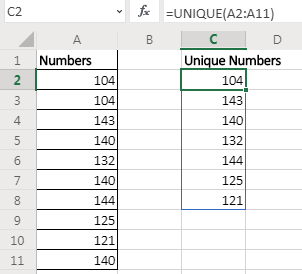As soon as you hit the enter button, the resultant array of unique value is spilled down the cells. This is a dynamic array that is only available for Excel 365 online.

Example 2: Extract Values that only occur once in the range.
In the above example, we got unique values from the range. If a value was occurring once, twice, or more we got only one stance of it. But if we want to extract values that only occurs once in the range (unique values in range) then the formula will be:

=UNIQUE(A2:A11,1)Here we set the exactly_once variable to TRUE. This returns the value that are unique in the range/array itself.

Example 3: Find Unique Values in Horizontal Data

In the above two examples, the array was vertical and had numeric values. What if we have non numeric values and horizontal data? Well, not to worry. The excel UNIQUE function works on any kind of value be it numeric, text, boolean, etc. And we can use it on horizontal data setup too.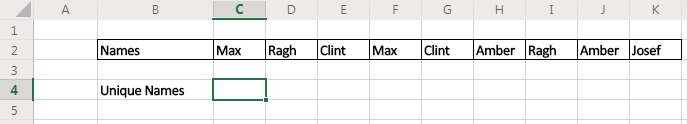Here I have some names in cells of adjacent columns. I want to get all names without repetition in the cells below.

The formula will be:

=UNIQUE(C2:K2,,1)

It returns the names after removing all duplicates from the array in columns.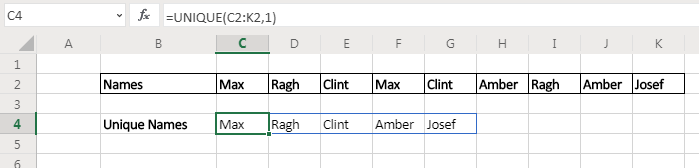So yeah guys, this is how the UNIQUE function of Excel 365 works. It is planned to be released on Excel 2019 pro version. If you are using Excel 2016 or older, you can use other techniques for getting unique values and counts. Below are the links.

Related Articles:

Excel Formula to Extract Unique Values From a List | To find all unique values from a list in Excel 2016 and older versions, we use this simple formula.

UNIQUE Function for Excel 2016 | The Excel 2016 does not provide the UNIQUE function but we can create one. This UDF UNIQUE function returns all unique values from the list.

How To Count Unique Values in Excel With Criteria | To count unique values in excel with criteria we use a combination of functions. The FREQUENCY function is core of this formula

Popular Articles:

50 Excel Shortcuts to Increase Your Productivity | Get faster at your task. These 50 shortcuts will make you work even faster on Excel.

How to use the VLOOKUP Function in Excel | This is one of the most used and popular functions of excel that is used to lookup value from different ranges and sheets.

How to use the COUNTIF function in Excel 2016 | Count values with conditions using this amazing function. You don't need to filter your data to count specific values. Countif function is essential to prepare your dashboard.

How to use the SUMIF Function in Excel | This is another dashboard essential function. This helps you sum up values on specific conditions.

Terms and Conditions of use

The applications/code on this site are distributed as is and without warranties or liability. In no event shall the owner of the copyrights, or the authors of the applications/code be liable for any loss of profit, any problems or any damage resulting from the use or evaluation of the applications/code.